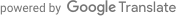## 數學筆記

$\tau_i = Y_i(1) - Y_i(0) \qquad(2.1)$

1 $$Y_1(1)$$ $$Y_1(0)$$ $$\tau_1$$
2 $$Y_2(1)$$ $$Y_2(0)$$ $$\tau_2$$
$$\vdots$$ $$\vdots$$ $$\vdots$$ $$\vdots$$
$$N$$ $$Y_N(1)$$ $$Y_N(0)$$ $$\tau_N$$

$\text{ATE} = \bar{\tau} = \frac{1}{N} \sum_{i=1}^N \tau_i \qquad(2.2)$

$\text{ATE} = \frac{1}{N} \sum_{i=1}^N Y_i(1) - \frac{1}{N} \sum_{i=1}^N Y_i(0) \qquad(2.3)$

$\widehat{\text{ATE}} = \underbrace{\frac{1}{N_t} \sum_{i:W_i=1} Y_i(1)}_{\text{average earnings, treatment}} - \underbrace{\frac{1}{N_c} \sum_{i:W_i=0} Y_i(0)}_{\text{average earnings, control}} \qquad(2.4)$

1 $$Y_1(0)$$
2 $$Y_2(1)$$
$$\vdots$$ $$\vdots$$ $$\vdots$$ $$\vdots$$
$$N$$ $$Y_N(1)$$

Defiers 不， $$W_i(Z_i=1) = 0$$ 是的， $$W_i(Z_i=0) = 1$$

$\text{ITT}_{W,i} = W_i(1) - W_i(0) \qquad(2.5)$

$\text{ITT}_{W} = \frac{1}{N} \sum_{i=1}^N [W_i(1) - W_i(0)] \qquad(2.6)$

$\widehat{\text{ITT}_{W}} = \bar{W}^{\text{obs}}_1 - \bar{W}^{\text{obs}}_0 \qquad(2.7)$

$\text{ITT}_{Y,i} = Y_i(1, W_i(1)) - Y_i(0, W_i(0)) \qquad(2.8)$

$\text{ITT}_{Y} = \frac{1}{N} \sum_{i=1}^N [Y_i(1, W_i(1)) - Y_i(0, W_i(0))] \qquad(2.9)$

$\widehat{\text{ITT}_{Y}} = \bar{Y}^{\text{obs}}_1 - \bar{Y}^{\text{obs}}_0 \qquad(2.10)$

$\text{CACE} = \frac{1}{N_{\text{co}}} \sum_{i:G_i=\text{co}} [Y(1, W_i(1)) - Y(0, W_i(0))] \qquad(2.11)$$\text{CACE} = \frac{\text{ITT}_Y}{\text{ITT}_W} \qquad(2.12)$

$\widehat{\text{CACE}} = \frac{\widehat{\text{ITT}_Y}}{\widehat{\text{ITT}_W}} \qquad(2.13)$